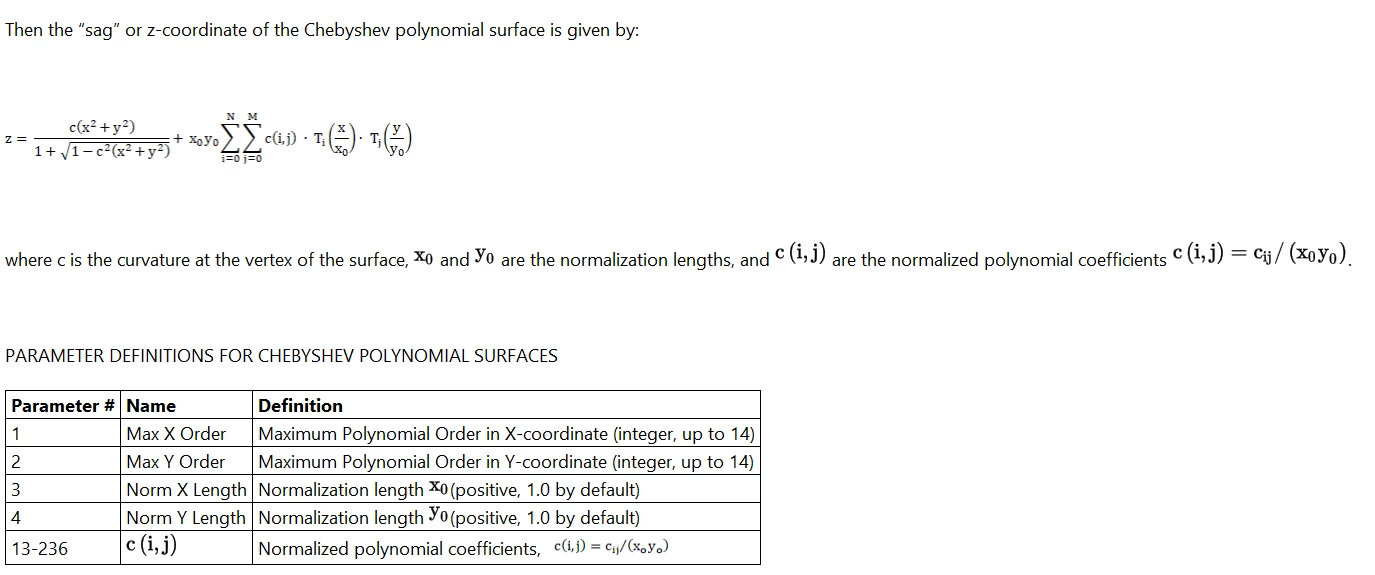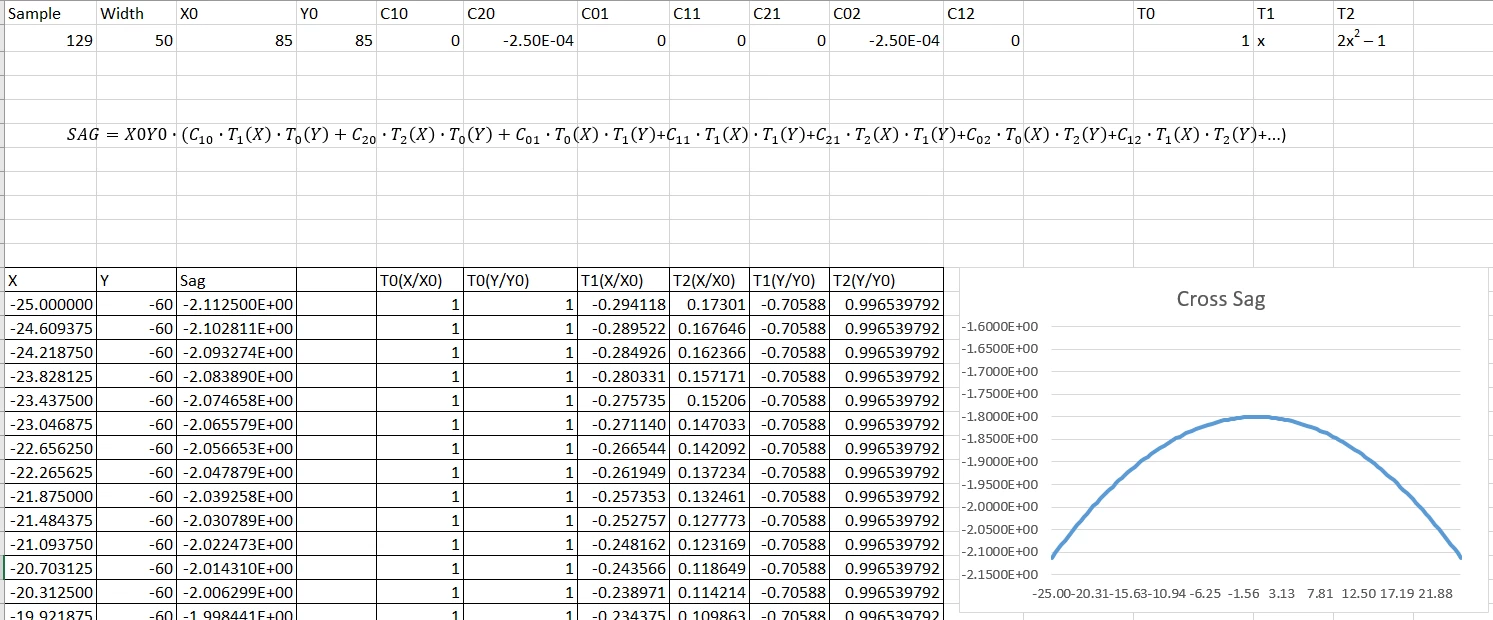# Understanding of coefficients of Chebyshev polynomial surface

• 3 replies
• 87 views

Userlevel 4+1
• Zemax Staff
• 41 replies
From the description in helpfile, coefficients used by OpticStudio is the Normalized polynomial coefficients, c(i,j)= cij/(x0y0), my question is how to connect these parameters with the final sag data?### 3 replies

Userlevel 4+1
For detailed discussion about Chebyshev surface, please refer to the KB article: Freeform Optics in OpticStudio

Check the attached excel file to compare computed sag data and the sag value used by OpticStudio.

The calculation is based on the sample file located at

C:\Users\...\Documents\Zemax\Samples\Sequential\Tilted systems & prisms\OAP using Chebyshev Polynomial surface.ZMXHi,

The calculation of T2(X/X0) should be 2*(X/X0)^2 -1, why the -1 is not considered? Same in the calculation of T2(Y/Y0)....

Could you check please? If the -1 is added, then there will be a strange thing that, in the center of the surface, the sag is not zero....
Userlevel 4+1

Hi Yunfeng,

The calculation is a comparison between the results in OpticStudio and the direct calculation with the polynomial. The constant term is not used in the calculation, which yields the same result with OpticStudio. I think the constant term can be considered by thickness of the surface or thickness of the preceding surface in sequential system. Let me know if you have further question about it.

Thanks.

Julia